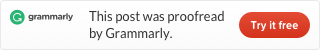Categories

# LeetCode 147 Insertion Sort List [sort] java

Sort a linked list using insertion sort.

A graphical example of insertion sort. The partial sorted list (black) initially contains only the first element in the list.
With each iteration one element (red) is removed from the input data and inserted in-place into the sorted list

Algorithm of Insertion Sort:

1. Insertion sort iterates, consuming one input element each repetition, and growing a sorted output list.
2. At each iteration, insertion sort removes one element from the input data, finds the location it belongs within the sorted list, and inserts it there.
3. It repeats until no input elements remain.

Example 1:

```Input: 4->2->1->3
Output: 1->2->3->4```

Example 2:

```Input: -1->5->3->4->0
Output: -1->0->3->4->5```

This is a textbook algorithm. It is not complex, we need a loop, then put list’s head as current. Continue the Loop unless current is null. We use the dummy as head of the new list.

Make next as current.next, use current to compare with node from dummy. Then continue the loop, Code like this: